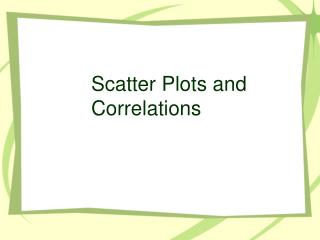DownloadDownload PresentationScatter Plots and Correlations

# Scatter Plots and Correlations

Télécharger la présentation## Scatter Plots and Correlations

- - - - - - - - - - - - - - - - - - - - - - - - - - - E N D - - - - - - - - - - - - - - - - - - - - - - - - - - -
##### Presentation Transcript

1. Scatter Plots and Correlations

2. Is there a relationship between the amount of gas put in a car and the number of miles that can be driven?

3. Positive Correlation • As one set of data values increases, so does the other.

4. Positive Correlation

5. Is there a relationship between the number of things you buy at the mall and the amount of money you have left?

6. Negative Correlation • As one set of data values increases, the other decreases.

7. Negative Correlation

8. Is there a relationship between a person’s age and the temperature outside?

9. No Correlation • There is no relationship between the two sets of data.

10. No Correlation

11. Would a scatter plot show a positive,negative, or no correlation? • Number of pages printed by a printer and the amount of ink left in the cartridge. • Negative Correlation!

12. Would a scatter plot show a positive,negative, or no correlation? • Age of a child and the child’s shoe size • Positive Correlation!

13. Would a scatter plot show a positive,negative, or no correlation? • Number of letters in a person’s first name and the person’s height. • No Correlation!

14. Would a scatter plot show a positive,negative, or no correlation? • Shots attempted and the number of points made in a basketball game • Positive Correlation!

15. Would a scatter plot show a positive,negative, or no correlation? • Length of a taxi ride and the amount of the fare • Positive Correlation!

16. Would a scatter plot show a positive,negative, or no correlation? • Outside temperature and the cost of air conditioning. • Positive Correlation!

17. Would a scatter plot show a positive,negative, or no correlation? • Miles ridden on a bicycle and the thickness of the tire tread • Negative Correlation!

18. Would a scatter plot show a positive,negative, or no correlation? • Temperature outside and the amount of clothing a person wears • Negative Correlation!

19. Think of some situations that show a…. • Positive Correlation: • Negative Correlation: • No Correlation:

20. Which graph to use…..? • Use bar graphs to make comparisons. • Use line graphs to show change over time. • Use circle graphs to show percentage of a whole. • Use histograms when the data is arranged in intervals. • Use scatter plots to show correlations between two sets of numerical data.

21. Using the appropriate measure of central tendency In a company there are 10 employees who each make \$10,000 per year. The boss makes \$80,000 per year. A job recruiter tells applicants that the average salary at the company is \$16,364. Is this misleading?

22. Which measure of central tendency did the job recruiter use? • Which measure of central tendency should he have used?

23. What measure of central tendency to use? Use the mean when the data values are close together. • Use the median when there are large gaps in the data values. • Use the mode when looking for the most common outcome.